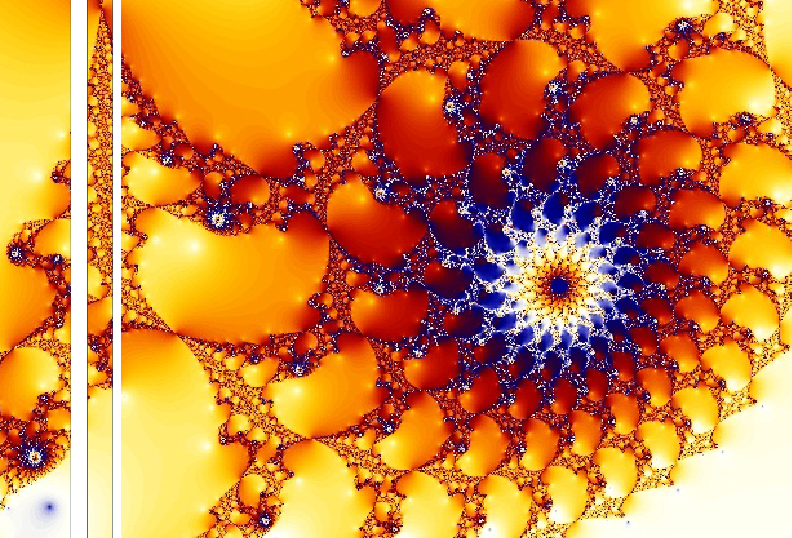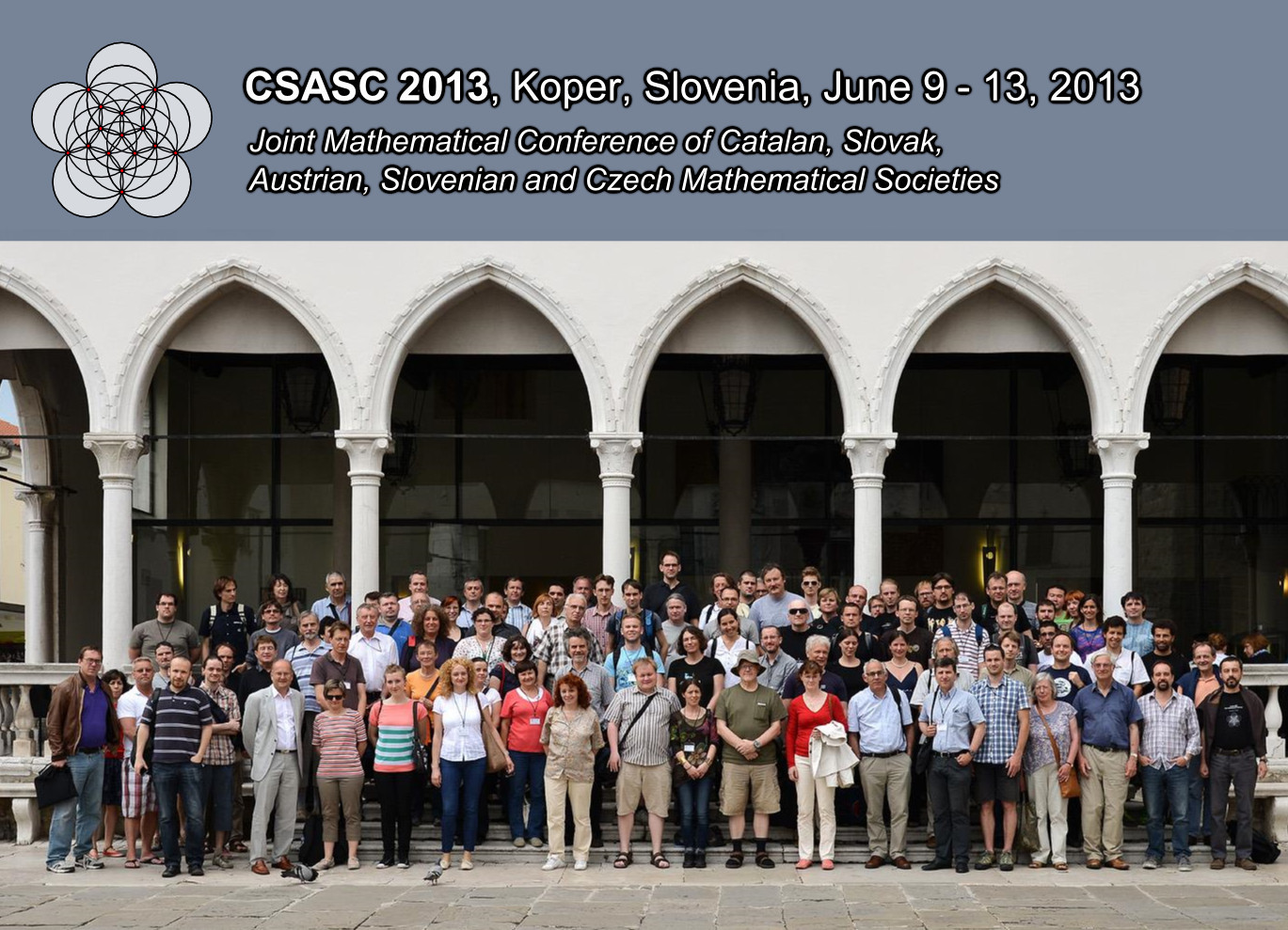Univerza na Primorskem Fakulteta za matematiko, naravoslovje in informacijske tehnologijeSI | EN

# Graph Theory Semester - Elena Valentinova Konstantinova

natisni

Combinatorial problems on Cayley graphs

The definition of Cayley graph was introduced by A.Cayley in 1878 to explain the concept of abstract groups which are generated by a set of generators in Cayley's time. In the last fifty years, the theory
of Cayley graphs has been developed to a rather big branch in graph theory. It has relations with many practical problems, and also with some classical problems in pure mathematics such as classification,
isomorphism or enumeration problems. There are problems related to Cayley graphs which are interesting to graph and group theorists such as hamiltonian or diameter problems, and to computer scientists and molecular biologists such as sorting by reversals. In this course we present some problems on Cayley graphs dealing with combinatorial and structural properties of graphs.

Lecturer: Elena Konstantinova, Sobolev Institute of Mathematics, Russia